Test Description

## 15 Questions MCQ Test Mathematics Olympiad for Class 9 | Math Olympiad Test: Quadrilaterals- 2

Math Olympiad Test: Quadrilaterals- 2 for Class 9 2022 is part of Mathematics Olympiad for Class 9 preparation. The Math Olympiad Test: Quadrilaterals- 2 questions and answers have been prepared according to the Class 9 exam syllabus.The Math Olympiad Test: Quadrilaterals- 2 MCQs are made for Class 9 2022 Exam. Find important definitions, questions, notes, meanings, examples, exercises, MCQs and online tests for Math Olympiad Test: Quadrilaterals- 2 below.
Solutions of Math Olympiad Test: Quadrilaterals- 2 questions in English are available as part of our Mathematics Olympiad for Class 9 for Class 9 & Math Olympiad Test: Quadrilaterals- 2 solutions in Hindi for Mathematics Olympiad for Class 9 course. Download more important topics, notes, lectures and mock test series for Class 9 Exam by signing up for free. Attempt Math Olympiad Test: Quadrilaterals- 2 | 15 questions in 15 minutes | Mock test for Class 9 preparation | Free important questions MCQ to study Mathematics Olympiad for Class 9 for Class 9 Exam | Download free PDF with solutions
 1 Crore+ students have signed up on EduRev. Have you?
Math Olympiad Test: Quadrilaterals- 2 - Question 1

### In a quadrilateral, the angles are in the ratio 1 : 2 : 3 : 4. What is the value of largest angle?

Detailed Solution for Math Olympiad Test: Quadrilaterals- 2 - Question 1

Let the angles of the quadrilateral be x°, 2x°, 3x° and 4x°
∵ Sum of the angles of quadrilateral = 360°
⇒ x + 2x + 3x + 4x = 360°
⇒10x = 360°
⇒ x = 36°
∵ Measure of largest angle
= 4 x x = 1 = 4 x 36° = 144°

Math Olympiad Test: Quadrilaterals- 2 - Question 2

### The perimeter of a parallelogram is 24 cm. If the longer side measures 8 cm. Then what is the measure of the shorter side?

Detailed Solution for Math Olympiad Test: Quadrilaterals- 2 - Question 2

Perimeter of parallelogram = 2(a + b) = 24 cm
⇒ a + b = 12 cm
Given a = 8 cm
then 8 + b = 12 cm
⇒ b = 4 cm

Math Olympiad Test: Quadrilaterals- 2 - Question 3

### ABCD is a square. What is the value of ∠ACD?Detailed Solution for Math Olympiad Test: Quadrilaterals- 2 - Question 3

∵ ABCD is a square
∴ ∠D = 90° and AD = DC = AB = BC
∴ ∠CAD = ∠ACD, and
∠D + ∠ACD + ∠CAD = 180°
⇒ 90° + 2 ∠ACD = 180°
⇒ ∠ACD = 90°​​​​​​​/2 = 45°

Math Olympiad Test: Quadrilaterals- 2 - Question 4

The figure formed by joining the mid-points of consecutive sides of a quadrilateral is a

Detailed Solution for Math Olympiad Test: Quadrilaterals- 2 - Question 4

The figure formed by joining the midpoints of consecutive side of a quadrilateral is a parallelogram.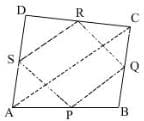Math Olympiad Test: Quadrilaterals- 2 - Question 5

The angles of a quadrilateral are in the ratio 2 : 4 : 5 : 7. What is the difference between largest and smallest angle?

Detailed Solution for Math Olympiad Test: Quadrilaterals- 2 - Question 5

Let the angles be 2x, 4x, 5x and 7x respectively.
∴ Difference between largest and smallest angle = 7x - 2x = 5x
∵ Sum of all angles of a quadrilateral = 360°
⇒ 2x + 4x + 5x + 7x = 360°
⇒ 18x = 360°
⇒ x = 20°
∴ Required difference
= 7x - 2x = 5x
= 5 x 20° = 100°

Math Olympiad Test: Quadrilaterals- 2 - Question 6

If an angle of a parallelogram is two third of its adjacent angle what is the measure of smallest angle of parallelogram?

Detailed Solution for Math Olympiad Test: Quadrilaterals- 2 - Question 6

Let the angle be x°
∴ Its adjacent angle = (180 - x)°
A/Q,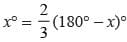⇒ 3x° = 360° - 2x°
⇒ 5x = 360°
⇒ x = 72°

Math Olympiad Test: Quadrilaterals- 2 - Question 7

If ∠P, ∠Q, ∠R, ∠S of a quadrilateral PQRS, taken in order are in the ratio 3 : 7 : 6 : 4, then PQRS is a

Detailed Solution for Math Olympiad Test: Quadrilaterals- 2 - Question 7

Rhombus, parallelogram, and kite have their opposite angles equal (i.e. in the ratio 1 : 1). Therefore, the quadrilateral PQRS is a trapezium.

Math Olympiad Test: Quadrilaterals- 2 - Question 8

The figure formed by joining the mid-points of the adjacent sides of a square is

Detailed Solution for Math Olympiad Test: Quadrilaterals- 2 - Question 8

P, Q, R and S are the mid-points of BA, BC, CD and DA respectively.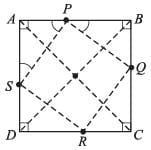∴ AP = AS = PB = BQ = QC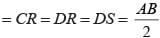∴ In ΔAPS
AP = AS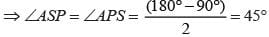Similarly
Similarly ∠ASP = ∠APS =∠BPQ = ∠BQP = ∠CQR
= ∠CRQ = ∠DSR = ∠DRS = 45°
Now ∠P + ∠ASP + ∠APS = 180°
⇒ ∠P = 90°
Similarly,
∠P = ∠Q = ∠R = ∠S = 90°
∴ PQRS is a parallelogram having each of its angles = 90°
Now,
using midpoint theorem in ΔABC and ΔACD
SR = PQ = 1/2 AC, and in ΔABD and ΔBDC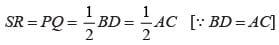∴ SP = PQ = QR = RS
∴ PQRS is a square

Math Olympiad Test: Quadrilaterals- 2 - Question 9

D is the mid-point of side AB of a parallelogram ABCD. A line through B parallel to PD meets DC at Q and AD produced at R, then which of the following is correct?

Detailed Solution for Math Olympiad Test: Quadrilaterals- 2 - Question 9

In ΔABR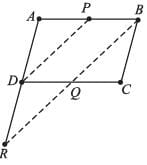DP ∥ BR, and P is the mid-point of side AB
∴ Using mid-point theorem (converse) Point P is the mid-point of AB and is parallel to BR
∴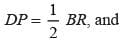D will be the mid-point of side AR
∴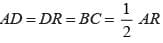⇒ AR = 2BC

Math Olympiad Test: Quadrilaterals- 2 - Question 10

If ABCD is a square then what is the measure of ∠DCA?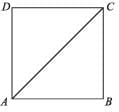Detailed Solution for Math Olympiad Test: Quadrilaterals- 2 - Question 10

∠DAC = ∠​​​​​​​DCA = 90°​​​​​​​/2 = 45°

Math Olympiad Test: Quadrilaterals- 2 - Question 11

Two opposite angles of a parallelogram are (3x - 2)° and (50 - x)°. Find the smallest angle.

Detailed Solution for Math Olympiad Test: Quadrilaterals- 2 - Question 11

∵ The opposite angles of a parallelogram are equal.
∴ (3x - 2)° = (50 - x)°
⇒  4x = 52°
⇒ x = 13°
∴ (3x - 2)° = 37°
∴ (50 - x)° = 37°

Math Olympiad Test: Quadrilaterals- 2 - Question 12

If an angle of a parallelogram is one third of its adjacent angle then what is the measure of smallest angle?

Detailed Solution for Math Olympiad Test: Quadrilaterals- 2 - Question 12

Let the angle be x°
∴ Adjacent angle = (180 - x)°
⇒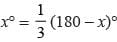⇒ 4x = 180°
⇒ x = 45°

Math Olympiad Test: Quadrilaterals- 2 - Question 13

The diagonals of a rectangle PQRS meet at O. If ∠SOR = 64° then Find ∠OAC?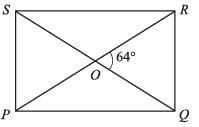Detailed Solution for Math Olympiad Test: Quadrilaterals- 2 - Question 13

∵ Diagonals of a rectangle bisect each other and are also equal in length.
∴ In ΔPOS,
OP = OS
⇒ ∠OPS = ∠OSP
(angles opposite to equal sides are equal)
Also,
∠POS + ∠OSP + ∠OPS = 180°
⇒ 2∠OPS = 180° - ∠POS
= 180° - 64° (∵ ∠POS + ∠QOR) {vertically opposite∠s}
⇒ ∠POS​​​​​​​ = 116°​​​​​​​/2 = 58°

Math Olympiad Test: Quadrilaterals- 2 - Question 14

The angles of a quadrilateral are 98°, 92°, 70° respectively. What is the measure of 4th angle?

Detailed Solution for Math Olympiad Test: Quadrilaterals- 2 - Question 14

Let the measure of 4th angle be x°
∴ 98° + 92° + 70° + x° = 180° × 2 = 360°
⇒ x° = 100°

Math Olympiad Test: Quadrilaterals- 2 - Question 15

If the length of each side of rhombus is 15 cm and one of its diagonals is 24 cm what is length of other diagonal?

Detailed Solution for Math Olympiad Test: Quadrilaterals- 2 - Question 15

∵ Diagonals of a rhombus bisect each other at 90°
In ΔAOD
AD = 15 cm, AC = 12 cm,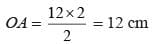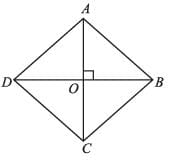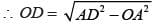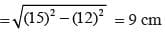∴ BD = 2OD
= 2 × 9 = 18 cm

## Mathematics Olympiad for Class 9

1 videos|43 tests
 Use Code STAYHOME200 and get INR 200 additional OFF Use Coupon Code